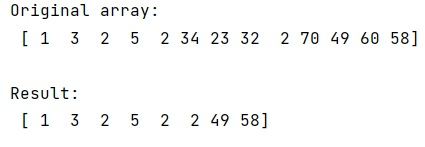# Python | NumPy where() Method with Multiple Conditions

Use numpy.where() with Multiple Conditions: In this tutorial, we will learn how to use multiple conditions with NumPy where() method in Python? By Pranit Sharma Last updated : April 17, 2023

## Using Multiple Conditions (OR (|), AND (&)) in numpy.where()

Suppose that we are given a numpy array that contains some numerical values and we need to extract values using multiple conditions using the OR operator.

For example, given array as [1, 3, 2, 5, 2, 70, 49, 60, 58] and we need to create another array as AREA whose values depend on the multiple conditions. This can be achieved with the help of `numpy.where()` method by using multiple conditions.

## How to use NumPy where()with multiple conditions?

To use `numpy.where()` with multiple conditions, just put the conditional expression having multiple conditions combined with logical OR (|) and logical AND (&) operators inside the `where()` method. It will return the result based on the given multiple conditions.

Let us understand with the help of an example,

## Python Program to Use NumPy where() Method with Multiple Conditions

```# Import numpy
import numpy as np

# Creating an array
arr = np.array([1,3,2,5,2,34,23,32,2,70,49,60,58])

# Display original array
print("Original array:\n",arr,"\n")

# Extracting values based on multiple conditions
res = np.where(((arr>0) & (arr<10)) | ((arr>40) & (arr<60)))

# Creating array AREA
AREA = arr[res]

# Display result
print("Result:\n",AREA)
```

### Output